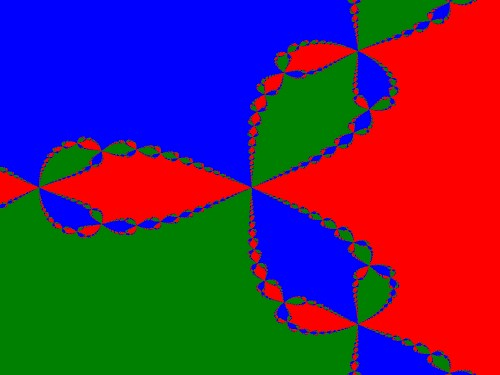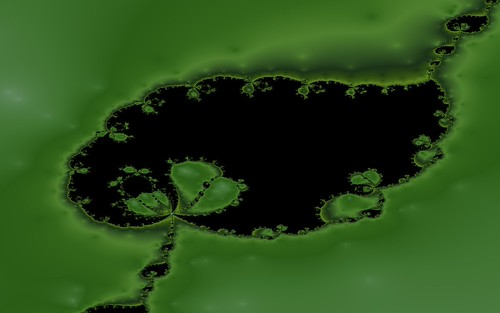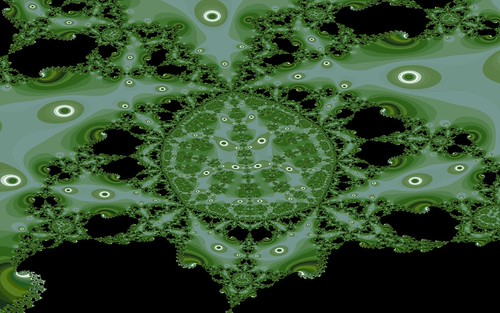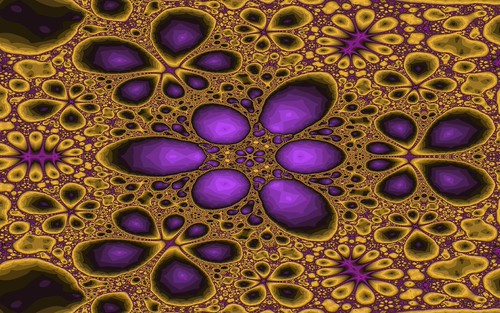# Root-Finding Fractals

Newton Fractals

You may have seen Newton fractals like the following image.The image comes from the formula z^3-1=0. When this fomula is plotted to the complex plane it has 3 distinct roots or possible values for z that satisfy the equation. Each “pixel” (or location in the 2D complex plane) of the image is set to the initial z complex number value at that coordinate and then iterated through the root finding method equation. The resulting pixel colors form a basin of attraction. Each pixel is colored red, green or blue depending on which root it finds. Points close to the target roots (or “zeroes”) are surrounded by a single color, but when there is an edge between two roots the surprisingly complex structures emerge. The process to find the roots is called Newton’s Method hence the name Newton Fractals.

A much more pleasing coloring method is to map how long it takes the iteration to reach a target root. The iteration count is mapped to a color palette and then plotted. This results in images like the following which uses the formula z^8+15z^4-16=0.To see more sample images visit my Flickr Newton Fractals gallery.

Since Newton many other mathematicians have devised other formulas to solve the problem of finding multiple roots to polynomial equations. These can also be used in a similar way to generating the Newton Fractals to create new and unique images.

Halley Fractals

Halley’s Method from Edmund Halley (yes, he did more than work out the orbit of that comet) gives Halley Fractals like the following samples.To see more sample images visit my Flickr Halley Fractals gallery.

Here is a sample movie of a Halley Fractal.

Schroder Fractals

Schroder’s Method gives some very nice results and they tend to be more complex looking and detailed than the Newton and Halley results.To see more sample images visit my Flickr Schroder Fractals gallery.

Here are two sample movies of Schroder Fractals.

Householder Fractals

Next up is Householder’s Method for root finding. This again gives unique results for the fractal images.To see more sample images visit my Flickr Householder Fractals gallery.

Here is a sample movie of a Householder Fractal.

Secant Fractals

Using the Secant Method is another way of creating root-finding fractal images.To see more sample images visit my Flickr Secant Fractals gallery.

Here are two sample movies of Secant Fractals.

Summary

There are a whole family of fractal images to be discovered by using these various methods to find the roots of polynomial equations. None of these methods were ever originally intended to be used for fractals, but thanks to the discoverers fractal enthusiasts have another wide range of images to explore.

Thanks go out to Florian Brucker and Simon Tatham whose websites helped me to get the hang of these formulas.

Special thanks must also go to the amazing WolframAlpha website that is a front end to the power of Mathematica which helped invaluably in finding roots, derivatives and second derivatives of the equations necessary to be able to create the above images. I have always been impressed with Mathematica when I have had a chance to use it but it is a rip off so I am glad that they decided to put the power into their online version.

All of the above images and videos were created with Visions Of Chaos.

Jason.

## 2 responses to “Root-Finding Fractals”

1.Lari says:

Wonderful and fascinating!
Cheers!